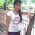# Math for Computer science Questions and Answers 281 to 290

## Math for Computer science

### Questions 281 to 290

 281 What is the 5th term of (3 + x)8 ? (a)  C(8, 4) 34 x4                                      (b)  C(8, 5) 33 x5       (c)  C(8, 4) 34 x5 (d)  C(8, 4) 35 x4                                     (e)  C(7, 4) 34 x4. 282 How many different strings can be made by reordering the letters of the word ABRACADABRA (a)  8 ! / 5!2!2!         (b)  11! / 5!2!2!         (c)  5!2!2! / 11!         (d)  5!2!2! / 8!          (e)  11!. 283 What is the value of k after the following code has been executed. k := 0           for i1 := 1 to n1               for  i2 := 1 to n2               for i2 := 1 to n2               :               :                 for  im := 1 to nm                      k := k + 1 (a)  n1 + n2 + n3 +….nm                           (b)  n1.n2.n3…………nm                     (c)  C(n+m-1, m) (d)  C(n,m)                                             (e)  P(n,m). 284 GCD of 24.35.72 and 24.32 is (a)  22.35.72              (b)  24.35.72              (c)  25.34.72              (d)  24.32.70              (e)  22.35.74. 285 How many solutions are there to the equation x1 + x2 +x3+x4 = 17 (a)  C(20,17)                                          (b)  C(20 , 3)                                          (c)  1140                        (d)  All the above                                   (e)  none of the above. 286 Fermat’s theorem states that If  p is prime and a is an integer not divisible by p, then (a)  ap–1  1 (mod p)                                                             (b)  ap  1 (mod p)                    (c)  ap–1  0 (mod p) (d)  ap–1  1 (mod p–1)                            (e)  ap  1 (mod p–1). 287 Warshall’s algorithm is used for finding the __________ of a relation. (a)  reflexive closure                                                             (b)  symmetric closure              (c)  transitive closure (d)  transpose                                        (e)  inverse. 288 Suppose that A is a subset if V*. Then the set consisting of concatenations of arbitrarily many strings from A represented by A* is called (a)  Symmetric closure                           (b)  Reflexive closure                   (c)  Kleene closure (d)  Finite closure                                   (e)  Finite state closure. 289 The finite-state machine with no output is called _________ (a)  Kleene machine                               (b)  Finite machine                (c)  Output-free machine (d)  Finite-state automata                        (e)  Moore machine. 290 Type 2 grammars are also called ­__________ (a)  Context-sensitive grammars             (b)  Regular grammars            c)  Context-free grammars (d)  Context less grammars                    (e)  Irregular grammars.

#### Answers

 281 Answer : (a) Reason : For finding the 5th term use the general term formula tr+1 = C(x, y)xn–r yr. Here you have to find the term tr+1. 282 Answer : (b) Reason : This is a problem of permutations with repetitions. If n be the total number of letters and r1 letters are of same type and r2 letters are of same type then the number of different strings by reordering the letters of the word is n!/r1! × r2! 283 Answer : (b) Reason : By the definition of product rule. 284 Answer : (d) Reason : Gcd of 24.35.72 and 24.32 is 2 min(4,4) 3 min(5,2) 7 min(2,0) = 24.32.70 285 Answer : (d) Reason : This is combinations with indistinguishable objects. Here n=4 and r=17. The number of solutions is equal to the number of 17-combinations with repletion allowed from  a set with 4 elements. Therefore the answer is C(4+17-1,17) = C(20,17) = C(20,3). 286 Answer : (a) Reason : Definition of Fermat’s theorem 287 Answer : (c) Reason : Warshall’s algorithm is one of the algorithms for finding the transitive closure of the relation. 288 Answer : (c) Reason : Definition of the kleene’s closure 289 Answer : (d) Reason : Definition of finite-state automata 290 Answer : (c) Reason : Type 2 grammars are also called context-free grammars.

#### 3 comments :

1.2.3.The article is great, it helps a lot for my English.
run 3